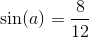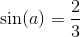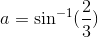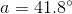# High School Math : Applying Trigonometric Functions

## Example Questions

### Example Question #1 : Triangles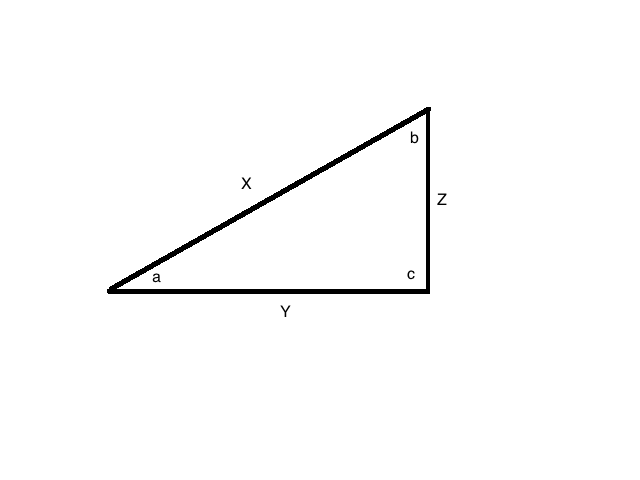In this figure, side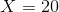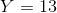, and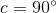. What is the value of angle?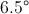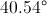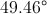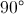UndefinedExplanation:

Since, we know we are working with a right triangle.

That means that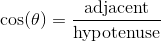.

In this problem, that would be: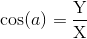Plug in our given values: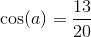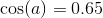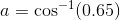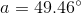### Example Question #2 : Triangles

Let ABC be a right triangle with sides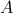= 3 inches,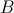= 4 inches, and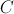= 5 inches. In degrees, what is the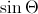where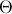is the angle opposite of side?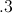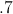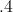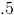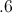Explanation: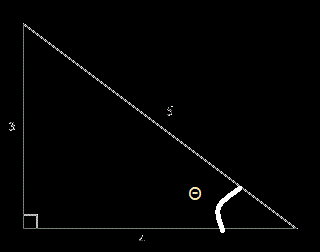We are looking for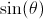. Remember the definition of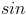in a right triangle is the length of the opposite side divided by the length of the hypotenuse.

So therefore, without figuring outwe can find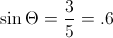### Example Question #5 : Graphing The Sine And Cosine FunctionsIn this figure, if angle, side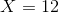, and side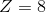, what is the measure of angle?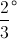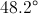Undefined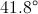Explanation:

Since, we know we are working with a right triangle.

That means that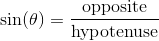.

In this problem, that would be: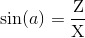Plug in our given values: